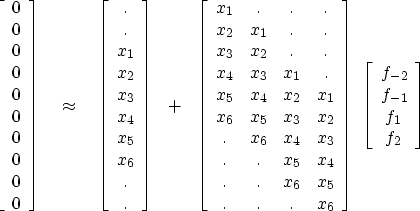Next: Stabilizing technique Up: Time-series analysis Previous: Setting up any weighted

# CALCULATING ERROR FILTERS

The error in prediction (or interpolation) is often more interesting than the prediction itself. When the predicted component is removed, leaving the unpredictable, the residual is the prediction error. Let us see how the program shaper() can be used to find an interpolation-error filter like (f-2,f-1,1,f1,f2). The statement of wishes is(22)
Taking the column vector of xt to the other side of the equation gives the form required by previous CG programs. After solving this system for (f-2,f-1,f1,f2), we insert the 1'' to make the IE filter

(f-2,f-1,1,f1,f2), which, applied to the data xt, gives the desired IE output.

Notice that the matrix in (22) is almost convolution. It would be convolution if the central column were not absent. I propose that you not actually solve the system (22). Instead I will show you a more general solution that uses the convolution operator itself. That way you will not need to write programs for the many almost'' convolution operators arising from the many PE and IE filters with their various gaps and lags.

The conjugate-gradient program here is a combination of earlier CG programs and the weighting methods we must introduce now: 1Next: Stabilizing technique Up: Time-series analysis Previous: Setting up any weighted
Stanford Exploration Project
10/21/1998